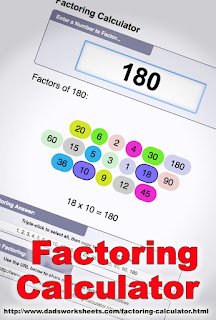### Visual tool - find all the factors of a numberHere's a nice factoring calculator that lists all the factors of a given number and also gives a neat VISUAL that pairs the factors.  You could use it to show your children/students some examples, and then of course let them PLAY with it! :)

See for example factors of 72, And all the factors of this large number.

Personally, I think it could be improved by listing the factors in order from 1 to the number itself, so that the pairing will then show how the largest and the smallest factors are paired, then the 2nd largest with the 2nd smallest, and so on. Neat anyway!